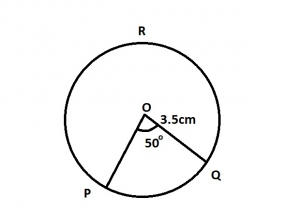In the diagram O is the center of circle PRQ. The radius is 3.5cm and ∠POQ = 50o. Use the diagram to answer the question below. (take π = 3.142). Calculate correct to one decimal place, the length of arc PQ.

A. 175.0cm
B. 157.1cm
C. 37.7cm
D. 11.0cm
E. 3.1cm